# A Satellite with Personality

August 7, 2015  -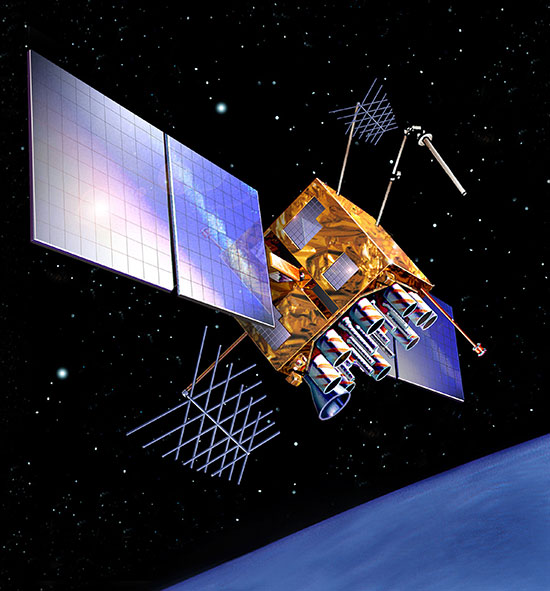SVN49 in space (artist’s rendering). The signal anomaly from SVN 49 alerted researchers to new possibilities in analysis and monitoring.

### Chip Transition-Edge Based Signal Tracking for Ultra-Precise GNSS Monitoring Applications

By Sanjeev Gunawardena, John Raquet and Frank van Graas

Tracking GNSS signals using their underlying spreading sequence chip transition edges reveals positive versus negative chip asymmetries that are characteristic to each satellite. This asymmetry is due to various types of natural signal deformation that is known to occur within the satellite’s signal generation and transmission hardware. This novel concept of monitoring chip asymmetry can extend the state of the art in the areas of GNSS signal-quality monitoring and authentication. A technique to directly monitor chip asymmetry within a specially designed ChipShape GNSS receiver architecture employs separate code discriminators that align themselves to the chip rising-edge and falling-edge zero crossings.

The detailed study of naturally-present deformations in GNSS signals is a relatively new activity that was sparked by the GPS SVN49 anomaly and the associated research activities that followed. This research area has numerous applications that include:

• Informing the design of sudden signal deformation detection and alerting algorithms for safety-of-life differential GNSS applications (such as aviation).
• GNSS signal “fingerprinting” and authentication.
• The detailed study of long-term degradation effects of GNSS satellite signal generation and transmission hardware.
• Analysis of the impact to the first item in this list of swapping a satellite’s signal generation modules by its control segment.

Multipath detection, characterization, and mitigation are also closely tied to all research relating to GNSS signal deformation monitoring (SDM).

High-fidelity SDM can be performed using two methods:

• observation of actual GNSS signals above the thermal noise floor using a high-gain dish antenna;
• the combination of long coherent integration and multi-correlator processing.

Our previous research has revealed that these two methods are highly complementary for gaining full insight into the effects and causes of observed natural signal deformations.

Among the handful of multi-correlator processing techniques that can be applied for SDM, ChipShape processing allows the correlation function resolution to be finely adjustable while providing good numerical processing efficiency. This processing technique also allows chip-transition eye diagrams to be constructed in order to provide additional insight such as positive and negative chip width asymmetries.

One goal of our SDM research involves developing capabilities to observe GNSS signals with the highest levels of fidelity practically achievable in order to further the application areas described above. Key to this is developing techniques to track GNSS signals using a reference point that is both consistent and invariant (to the greatest extent possible) to nominal signal deformations and environmental effects such as multipath. Traditional multipath mitigating techniques such as narrow correlator and double-delta correlator are sub-optimal in this regard. This is because a significant portion of the signal around the chip transition point (that is, 10 percent and 20 percent for 0.1 chip correlator spacing, respectively) must be integrated to realize these discriminators and maintain robust tracking in moderate dynamics conditions. This integration tends to low-pass filter the desired observables.

#### Chip Transition Edge-Based Code Tracking

Figure 1 illustrates normalized C/A code chip rising edges for the GPS constellation of June 2014. These chip shapes were processed using a front-end with 24 MHz bandwidth. For visual comparison purposes, this and other related plots were obtained using 600 seconds of coherent integration.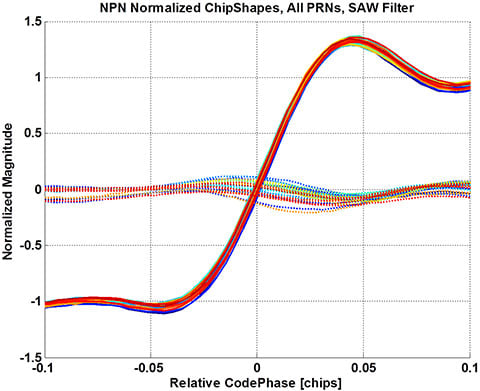Figure 1. Normalized ChipShape rising edges for the GPS SPS constellation of June 2014; each color represents a different GPS satellite.

The code tracking loop used to obtain this result employed an empirical normalized coherent rising-edge discriminator given by:

Where τ is relative code phase in chips, d is Early-Late correlator spacing,R’XYZ(i) is the differential correlation output for integer bin i obtained using ChipShape processing with masking sequence XYZ. bin(x) is a function that selects the closest ChipShape vector index that corresponds to relative code phase x. Each ChipShape processing bank is configured to span one chip early and one chip late with a resolution of N bins per chip, thus producing a ChipShape vector of 3N bins. α is a scale factor obtained through trial and error to yield robust tracking performance as observed by the code-minus-phase measurement. For the result shown in Figure 1, N=240 and d ≈ 0.017 chips.

The figure clearly shows that the rising-edge zero crossings vary by SV. This variation is due to nominal signal deformation present in each GPS-SPS signal.

Figure 2 illustrates the rising-edge zero crossings aligned to zero relative code phase. This alignment was performed by interpolating each R’NPN vector, precisely estimating code phase at the zero-crossing point, and shifting the curve appropriately.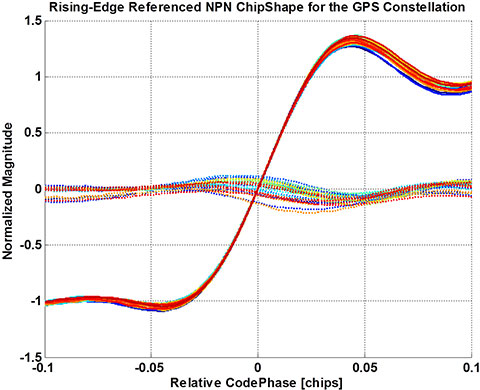Figure 2. Normalized ChipShape rising edges for the GPS SPS constellation of June 2014: Zero crossing compensated.

Figure 3 shows zero crossings for the falling edges after all rising edges were aligned to zero. The figure clearly illustrates subtle asymmetries between positive and negative chips which span a range of approximately ±1.5 meters. These asymmetries are not directly observable using typical GNSS receiver processing. However, they can lead to pseudorange biases through the resulting distortion that occurs to the traditional correlation function.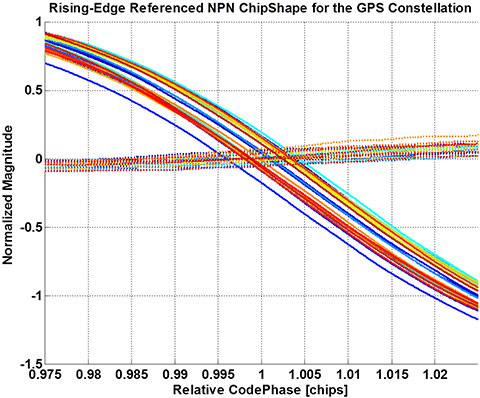Figure 3. Normalized ChipShape falling edges for the GPS SPS Constellation of June 2014 when rising edges are aligned to zero.

In general, a family of code discriminators that precisely track chip rising-edge zero crossings can be defined by:

Where R’NPX is a linear combination of orthogonal ChipShape components that preserve the rising-edge transition, e.g.: R’NPX = R’NPN + R’NPP. R’FFX is a linear combination of orthogonal ChipShape components that preserve the non-transitioning (that is, flat) sections of chips, for example: R’FFX = R’PPP + R’PPN R’NNP R’NNNa and b define an integration interval within the range −1 to +2 chips with respect to the chip transition edge. β is a bias compensation term.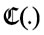represents the real or imaginary component function for the coherent discriminator (depending on the modulation phase of the signal being tracked), or the magnitude function for a non-coherent discriminator implementation.

Similarly, a family of code discriminators that precisely track chip falling-edge zero crossings that occur one chip after the rising edges tracked by the discriminator of Equation 2 can be defined by:

Then, a two-step technique to precisely monitor chip asymmetry can be described as follows:

• Setup two identical ChipShape processing channels to track a given PRN. Progressively tighten the code tracking loops to track the rising-edge zero crossings of the underlying signal using the discriminator of Equation 2.
• After steady-state zero-crossing rising-edge tracking is achieved, switch the second channel’s code discriminator to that of Equation 3. This will cause the second channel to track the zero crossings of the falling edges that occur one chip later in the underlying signal’s spreading sequence. The discriminator’s linear range must be wide enough to pull-in the chip asymmetry shown in Figure 3.

When the second channel re-converges as a result of Step 2, the relative pseudorange displacement that occurs is equal to the chip asymmetry in meters. Hence, chip asymmetry can be monitored for the entire visible pass of a satellite. It is expected that positive and negative chip transitions are equally affected by channel distortions (that is, code and carrier multipath, ionosphere, troposphere, and the receiver antenna and front-end transfer function). Hence, the rising-edge-code-minus-falling-edge-code measure of chip asymmetry is expected to be invariant to most if not all channel distortions.

#### Estimating Compensation Parameters

As shown in Equations 2 and 3, due to natural signal deformation of many types, the rising and falling-edge zero-crossing discriminators are expected to be SV number, PRN code and elevation angle dependent. Hence, α and β must be estimated for a given correlator spacing d separately for all SV signals of the constellation. These values will also be specific to a given antenna and receiver front-end.

Figure 4 illustrates the procedure used to estimate the scale factor and bias terms starting with the empirical rising-edge tracking process described above.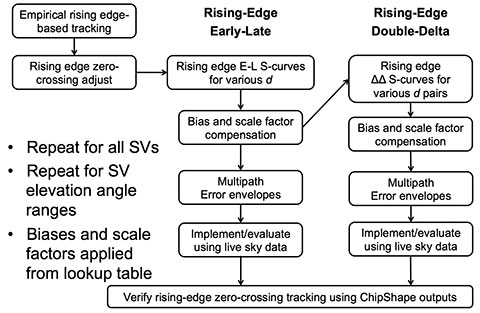Figure 4. Procedure for estimating scale factors and biases for rising-edge tracking early-late and double-delta code discriminators.

The following figures illustrate the edge tracking discriminator calibration process using R’NPN for a single SV.

Figure 5 illustrates the early-plus-late functions computed for various correlator spacings. As described previously, these functions typically do not cross through zero codephase due to natural signal deformation.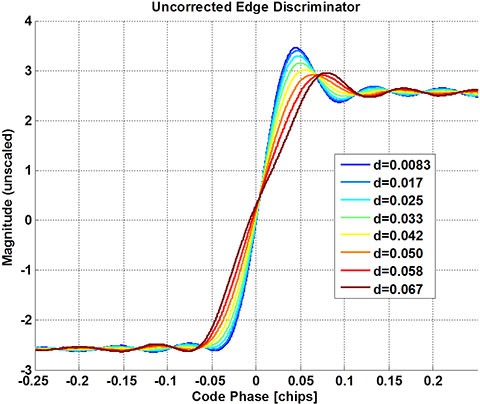Figure 5. Uncorrected rising-edge early-late discriminator functions for various correlator spacings.

Figure 6 illustrates the rising-edge discriminator functions after bias compensation.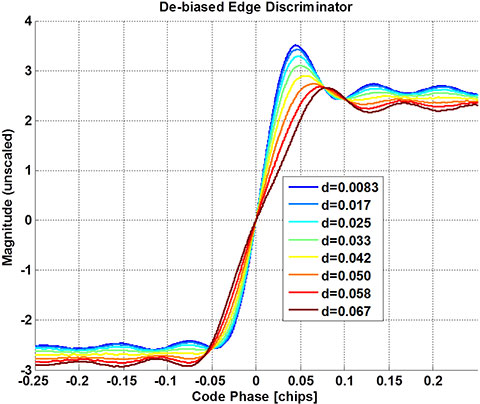Figure 6. Rising-edge early-late discriminator functions for various correlator spacings after bias compensation.

Figure 7 shows the fully calibrated Early-Late rising-edge tracking code discriminators.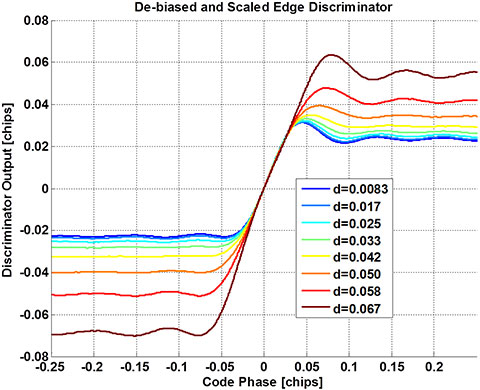Figure 7. Calibrated rising-edge early-late discriminator functions for various correlator spacings.

Figure 8 illustrates the multipath error envelopes for the rising edge-based coherent code discriminators. The performance of these discriminators is similar to the traditional Early-Late discriminators for the same correlator spacings. This result is consistent with the theoretical bounds for code multipath.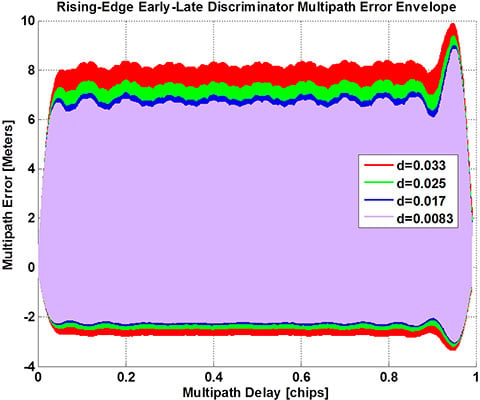Figure 8. Multipath error envelopes for various rising edge-based coherent early-late code discriminator functions.

As shown in Figure 4, the edge-tracking discriminators described in Equations 2 and 3 that are based on Early-Late bin spacings can be combined to obtain edge-tracking double-delta discriminators. Double-delta discriminators provide significantly improved multipath performance.

In general, the edge-tracking double-delta discriminator for inner correlator spacing d is formed by the linear combination of two early-late edge-tracking discriminators, as follows:

Scale factor γ is estimated such that overall multipath error is minimized according to a given design criteria.

Figure 9 illustrates the double-delta rising-edge discriminator with inner spacing of 0.017 chips. This discriminator has a pull-in range of approximately ±0.01 C/A chips.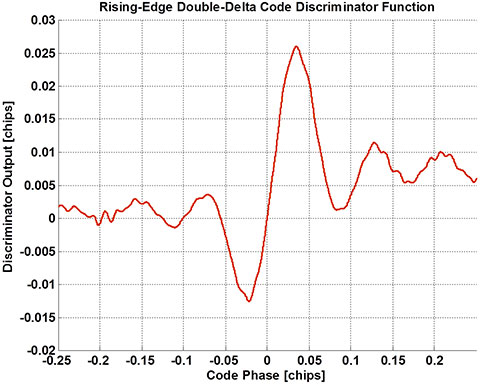Figure 9. Rising-edge coherent double-delta code discriminator function. Inner correlator spacing is ~0.017 C/A chips.

Figure 10 illustrates the non-linearity of this double-delta discriminator.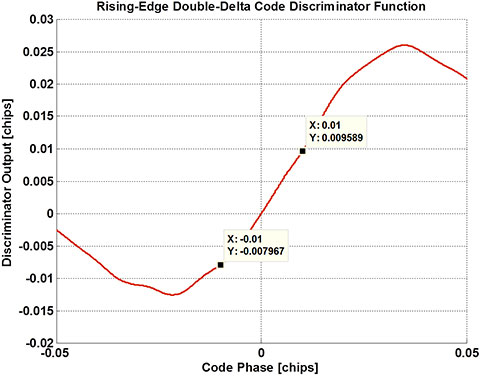Figure 10. Rising-edge coherent double-delta code discriminator function: Markers illustrate non-linearity.

Figure 11 illustrates the multipath error envelope for the coherent rising-edge double-delta discriminator. Performance is consistent with a traditional second-derivative discriminator.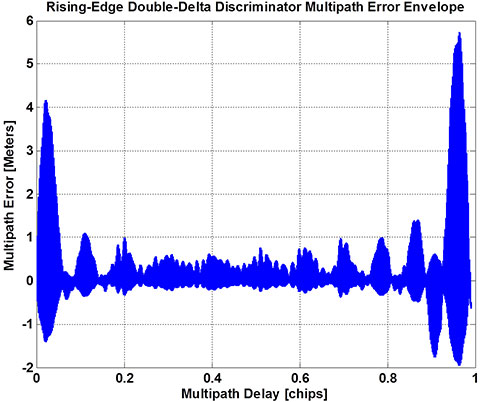Figure 11. Multipath error envelope for coherent rising-edge double-delta code discriminator with inner spacing of ~0.017 C/A chips.

Figure 12 illustrates the performance of the various rising-edge tracking discriminators for a live-sky GPS-SPS signal (de-trended code-minus-carrier measurement). This figure clearly demonstrates robust code tracking and the multipath and noise mitigating benefit of ultra-narrow rising-edge discriminators.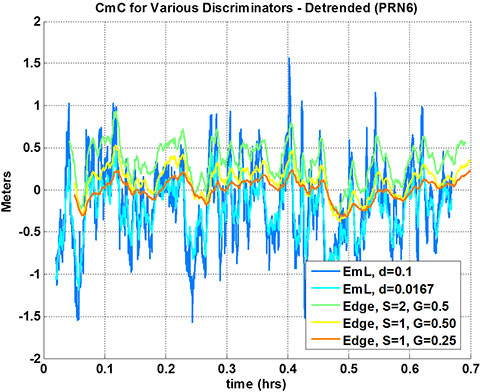Figure 12. Code tracking performance for live sky data of various rising edge-based coherent early-late code discriminator functions.

#### Conclusions

An empirical chip rising edge-based tracking technique was used to observe the underlying chip shapes of live sky GPS-SPS signals at high fidelity. These results reveal positive versus negative chip asymmetries that are characteristic to each satellite. The novel concept and technique of directly monitoring chip asymmetry has potential to extend the state of the art in the areas of GNSS signal quality monitoring and authentication.

Disclaimers. The views expressed in this paper are those of the authors and do not reflect the official policy or position of the United States Air Force, Department of Defense, or the United States Government.

Acknowledgments. This research was supported by the Air Force Research Laboratory Sensors Directorate.
The authors thank Ohio University Avionics Engineering Center for making available a cluster of high-performance computers to process the 20 TB dataset for this research, and Kadi Merbouh of Ohio University for maintaining and overseeing operation of this equipment.

The ChipShape processing is an extension of the signal compression technique first published by Larry Weill and licensed by NovAtel for use in its Vision Correlator technology.